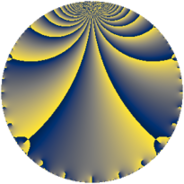# Properties

 Label 576.2.a.eLevel $576$ Weight $2$ Character orbit 576.a Self dual yes Analytic conductor $4.599$ Analytic rank $1$ Dimension $1$ CM discriminant -3 Inner twists $2$

# Related objects

Show commands: Magma / PariGP / SageMath

## Newspace parameters

comment: Compute space of new eigenforms

[N,k,chi] = [576,2,Mod(1,576)]

mf = mfinit([N,k,chi],0)

lf = mfeigenbasis(mf)

from sage.modular.dirichlet import DirichletCharacter

H = DirichletGroup(576, base_ring=CyclotomicField(2))

chi = DirichletCharacter(H, H._module([0, 0, 0]))

N = Newforms(chi, 2, names="a")

//Please install CHIMP (https://github.com/edgarcosta/CHIMP) if you want to run this code

chi := DirichletCharacter("576.1");

S:= CuspForms(chi, 2);

N := Newforms(S);

 Level: $$N$$ $$=$$ $$576 = 2^{6} \cdot 3^{2}$$ Weight: $$k$$ $$=$$ $$2$$ Character orbit: $$[\chi]$$ $$=$$ 576.a (trivial)

## Newform invariants

comment: select newform

sage: f = N # Warning: the index may be different

gp: f = lf \\ Warning: the index may be different

 Self dual: yes Analytic conductor: $$4.59938315643$$ Analytic rank: $$1$$ Dimension: $$1$$ Coefficient field: $$\mathbb{Q}$$ Coefficient ring: $$\mathbb{Z}$$ Coefficient ring index: $$1$$ Twist minimal: no (minimal twist has level 36) Fricke sign: $$1$$ Sato-Tate group: $N(\mathrm{U}(1))$

## $q$-expansion

comment: q-expansion

sage: f.q_expansion() # note that sage often uses an isomorphic number field

gp: mfcoefs(f, 20)

 $$f(q)$$ $$=$$ $$q - 4 q^{7}+O(q^{10})$$ q - 4 * q^7 $$q - 4 q^{7} - 2 q^{13} - 8 q^{19} - 5 q^{25} - 4 q^{31} + 10 q^{37} - 8 q^{43} + 9 q^{49} - 14 q^{61} + 16 q^{67} - 10 q^{73} - 4 q^{79} + 8 q^{91} + 14 q^{97}+O(q^{100})$$ q - 4 * q^7 - 2 * q^13 - 8 * q^19 - 5 * q^25 - 4 * q^31 + 10 * q^37 - 8 * q^43 + 9 * q^49 - 14 * q^61 + 16 * q^67 - 10 * q^73 - 4 * q^79 + 8 * q^91 + 14 * q^97

## Embeddings

For each embedding $$\iota_m$$ of the coefficient field, the values $$\iota_m(a_n)$$ are shown below.

For more information on an embedded modular form you can click on its label.

comment: embeddings in the coefficient field

gp: mfembed(f)

Label $$\iota_m(\nu)$$ $$a_{2}$$ $$a_{3}$$ $$a_{4}$$ $$a_{5}$$ $$a_{6}$$ $$a_{7}$$ $$a_{8}$$ $$a_{9}$$ $$a_{10}$$
1.1
 0
0 0 0 0 0 −4.00000 0 0 0
 $$n$$: e.g. 2-40 or 990-1000 Significant digits: Format: Complex embeddings Normalized embeddings Satake parameters Satake angles

## Atkin-Lehner signs

$$p$$ Sign
$$2$$ $$1$$
$$3$$ $$1$$

## Inner twists

Char Parity Ord Mult Type
1.a even 1 1 trivial
3.b odd 2 1 CM by $$\Q(\sqrt{-3})$$

## Twists

By twisting character orbit
Char Parity Ord Mult Type Twist Min Dim
1.a even 1 1 trivial 576.2.a.e 1
3.b odd 2 1 CM 576.2.a.e 1
4.b odd 2 1 576.2.a.f 1
8.b even 2 1 36.2.a.a 1
8.d odd 2 1 144.2.a.a 1
12.b even 2 1 576.2.a.f 1
16.e even 4 2 2304.2.d.q 2
16.f odd 4 2 2304.2.d.a 2
24.f even 2 1 144.2.a.a 1
24.h odd 2 1 36.2.a.a 1
40.e odd 2 1 3600.2.a.e 1
40.f even 2 1 900.2.a.g 1
40.i odd 4 2 900.2.d.b 2
40.k even 4 2 3600.2.f.m 2
48.i odd 4 2 2304.2.d.q 2
48.k even 4 2 2304.2.d.a 2
56.e even 2 1 7056.2.a.bb 1
56.h odd 2 1 1764.2.a.e 1
56.j odd 6 2 1764.2.k.g 2
56.p even 6 2 1764.2.k.h 2
72.j odd 6 2 324.2.e.c 2
72.l even 6 2 1296.2.i.h 2
72.n even 6 2 324.2.e.c 2
72.p odd 6 2 1296.2.i.h 2
88.b odd 2 1 4356.2.a.g 1
104.e even 2 1 6084.2.a.i 1
104.j odd 4 2 6084.2.b.f 2
120.i odd 2 1 900.2.a.g 1
120.m even 2 1 3600.2.a.e 1
120.q odd 4 2 3600.2.f.m 2
120.w even 4 2 900.2.d.b 2
168.e odd 2 1 7056.2.a.bb 1
168.i even 2 1 1764.2.a.e 1
168.s odd 6 2 1764.2.k.h 2
168.ba even 6 2 1764.2.k.g 2
264.m even 2 1 4356.2.a.g 1
312.b odd 2 1 6084.2.a.i 1
312.y even 4 2 6084.2.b.f 2

By twisted newform orbit
Twist Min Dim Char Parity Ord Mult Type
36.2.a.a 1 8.b even 2 1
36.2.a.a 1 24.h odd 2 1
144.2.a.a 1 8.d odd 2 1
144.2.a.a 1 24.f even 2 1
324.2.e.c 2 72.j odd 6 2
324.2.e.c 2 72.n even 6 2
576.2.a.e 1 1.a even 1 1 trivial
576.2.a.e 1 3.b odd 2 1 CM
576.2.a.f 1 4.b odd 2 1
576.2.a.f 1 12.b even 2 1
900.2.a.g 1 40.f even 2 1
900.2.a.g 1 120.i odd 2 1
900.2.d.b 2 40.i odd 4 2
900.2.d.b 2 120.w even 4 2
1296.2.i.h 2 72.l even 6 2
1296.2.i.h 2 72.p odd 6 2
1764.2.a.e 1 56.h odd 2 1
1764.2.a.e 1 168.i even 2 1
1764.2.k.g 2 56.j odd 6 2
1764.2.k.g 2 168.ba even 6 2
1764.2.k.h 2 56.p even 6 2
1764.2.k.h 2 168.s odd 6 2
2304.2.d.a 2 16.f odd 4 2
2304.2.d.a 2 48.k even 4 2
2304.2.d.q 2 16.e even 4 2
2304.2.d.q 2 48.i odd 4 2
3600.2.a.e 1 40.e odd 2 1
3600.2.a.e 1 120.m even 2 1
3600.2.f.m 2 40.k even 4 2
3600.2.f.m 2 120.q odd 4 2
4356.2.a.g 1 88.b odd 2 1
4356.2.a.g 1 264.m even 2 1
6084.2.a.i 1 104.e even 2 1
6084.2.a.i 1 312.b odd 2 1
6084.2.b.f 2 104.j odd 4 2
6084.2.b.f 2 312.y even 4 2
7056.2.a.bb 1 56.e even 2 1
7056.2.a.bb 1 168.e odd 2 1

## Hecke kernels

This newform subspace can be constructed as the intersection of the kernels of the following linear operators acting on $$S_{2}^{\mathrm{new}}(\Gamma_0(576))$$:

 $$T_{5}$$ T5 $$T_{7} + 4$$ T7 + 4 $$T_{11}$$ T11

## Hecke characteristic polynomials

$p$ $F_p(T)$
$2$ $$T$$
$3$ $$T$$
$5$ $$T$$
$7$ $$T + 4$$
$11$ $$T$$
$13$ $$T + 2$$
$17$ $$T$$
$19$ $$T + 8$$
$23$ $$T$$
$29$ $$T$$
$31$ $$T + 4$$
$37$ $$T - 10$$
$41$ $$T$$
$43$ $$T + 8$$
$47$ $$T$$
$53$ $$T$$
$59$ $$T$$
$61$ $$T + 14$$
$67$ $$T - 16$$
$71$ $$T$$
$73$ $$T + 10$$
$79$ $$T + 4$$
$83$ $$T$$
$89$ $$T$$
$97$ $$T - 14$$